178首页  > 魔兽世界  > 魔兽世界9.0暗影国度毁灭术大秘境的定位以及心得

# 魔兽世界9.0暗影国度毁灭术大秘境的定位以及心得

天赋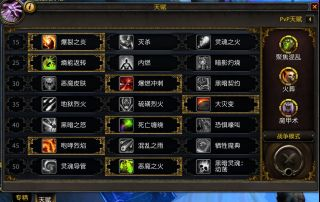然后说下这套天赋的特点和对本版本大秘境的理解

1.伤害平稳，即便没有爆发的情况下，单体和AOE也不会弱

2.因为不依赖爆发，所以也不太看重节奏

3.功能性不错 传送门、战复、群晕、(这个版本不能白嫖了)、战斗驱散、驱散队友有害魔法

因为机制的关系， 术士在高层大米是不可能打C位的， 如果说法师是C位， 那么术士和狂徒贼的伤害性质就非常类似，在FS萎靡期来补充伤害

可以理解为伤害性质上的互补， 也可以理解为 两个辅助配一个C位

正式基于此理解，这个版本我基本放弃了追求爆发流以及爆发峰值

找寻自身的定位，以及存在的价值，便不会让你觉得这个版本有多大的不平衡

=​=​=​=​=​=​=​=​=​=​=​=​=​=​=​=​=​=​=​=​=​=​=​=​=​=​=​=​=​=​=​=​=​=​=​=​=​=​=​=​=​=​=​=​=​=​=​=​=​=​=​=​=​=​=​=​=​=​=​=​=​=​=​=​=​=​=​=​=​=​=​=​=​=​=​=​=​=​=​=​=​=​

橙装选择

协同或燃烧

理由就是，这两个橙可以在几乎不动输出节奏的情况下稳定提升伤害

=​=​=​=​=​=​=​=​=​=​=​=​=​=​=​=​=​=​=​=​=​=​=​=​=​=​=​=​=​=​=​=​=​=​=​=​=​=​=​=​=​=​=​=​=​=​=​=​=​=​=​=​=​=​=​=​=​=​=​=​=​=​=​=​=​=​=​=​=​=​=​=​=​=​=​=​=​=​=​=​=​=​

盟约以及导灵器就拿最常用的法夜来说，目前因为盟约还没满，只能根据模拟数据来考虑，最终我大概率会选择尼亚，虽然第3个柯莱恩在模拟中排名第一， 但是柯莱恩单体羸弱

而这个版本的大米是非常讲究单体的，而尼亚的林地复兴(稳定提升各场合) 最终的锉刀(单体第一)更加适合

导灵器方面，最终尼亚要点出锉刀，导致只能上一个导灵器，基本只能选灰烬残骸

不过25级的时候尼亚开放第7排， 可以临时多用一个导灵器， 我应该会选择 噬者，提高灵魂腐化伤害(这个毕竟只是过渡，随便了~！)

=​=​=​=​=​=​=​=​=​=​=​=​=​=​=​=​=​=​=​=​=​=​=​=​=​=​=​=​=​=​=​=​=​=​=​=​=​=​=​=​=​=​=​=​=​=​=​=​=​=​=​=​=​=​=​=​=​=​=​=​=​=​=​=​=​=​=​=​=​=​=​=​=​=​=​=​=​=​=​=​=​=​

装备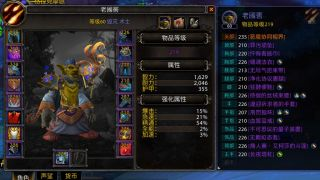关于属性 个人理解毁灭需要水桶腰(就是暴击急速精通都要稳步提高，不要偏属性) 说简单点，先提升装等， 属性优先用项链戒指这种不带智力的部位来调整

饰品方面， 既然不追求爆发，那么可以带双被动， 例如 团本尾王的赞美诗+无羁拟态者 或者选择我这套搭配， 三问号的CD正好配合地狱火(赞美诗我没有)

=​=​=​=​=​=​=​=​=​=​=​=​=​=​=​=​=​=​=​=​=​=​=​=​=​=​=​=​=​=​=​=​=​=​=​=​=​=​=​=​=​=​=​=​=​=​=​=​=​=​=​=​=​=​=​=​=​=​=​=​=​=​=​=​=​=​=​=​=​=​=​=​=​=​=​=​=​=​=​=​=​=​

手法方面我觉得没太多好说的，大家可以参考下我的录像或者看看其他人的帖子

我能说的就是，大秘境关键是把流畅度打出来， 不要过于考虑技能前后顺序(大体没错就行了，因为你总会有小失误)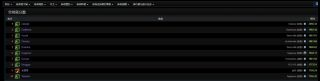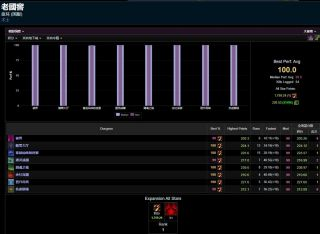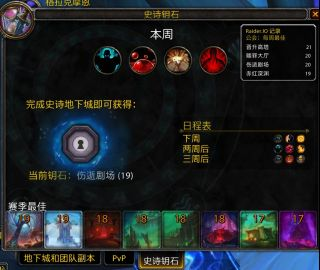178游戏网声明：登载此文出于传递更多信息之目的，并不意味着赞同其观点或证实其描述。

• 魔兽8.25
• 魔兽8.2
• 魔兽8.15
• 魔兽8.1
• 魔兽8.0
• 团队副本
• 常用工具